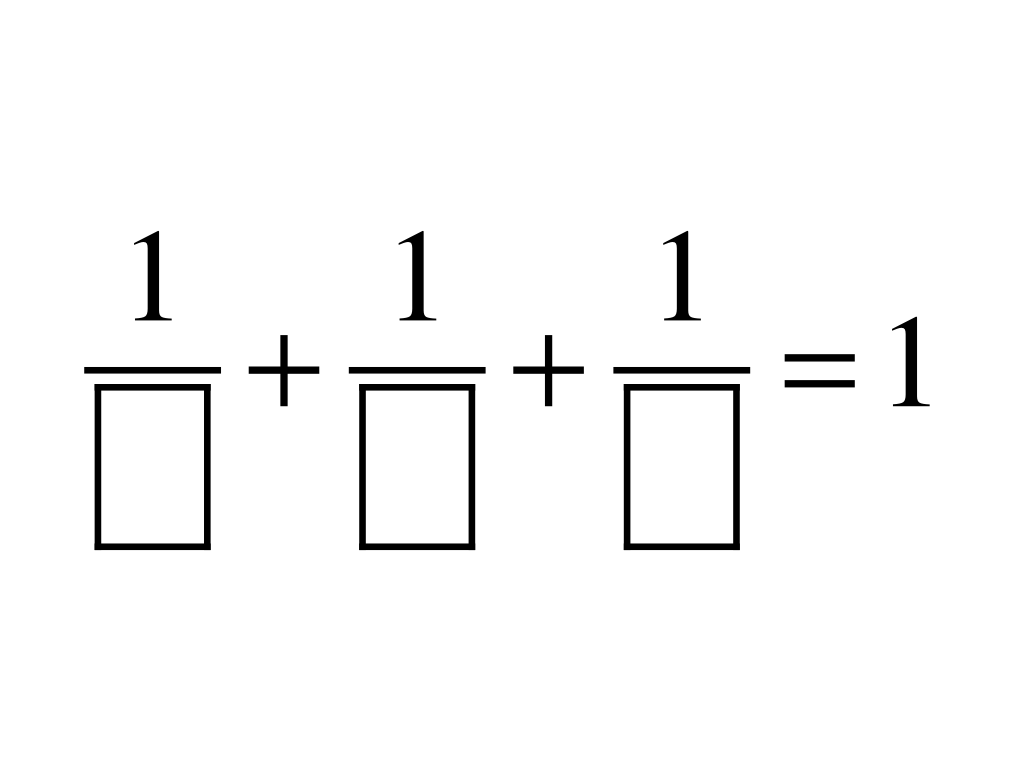# Sum to One

Strengthen your students’ facility with fractions through this playful challenge:### Challenges

1. Find three unit fractions whose sum is one.
2. How many different sets of three unit fractions can you find that have a sum of one?
3. How many different sets of four unit fractions can you find that have a sum of one?

### Setting

A unit fraction is any fraction of the form 1/n, where n is a positive integer. Many people have posed interesting questions about unit fractions over the years. While nothing in particular comes to mind, I am sure Martin Gardner’s work provided significant inspiration for this lesson, even if only indirectly. (You can read more about unit fractions here.)

With its low floor and high ceiling, this task is appropriate for students as young as Grade 4 and as old as Grade 12 (and likely beyond).

### Lesson Notes

Begin by displaying this image:Ask students to complete the unit fractions to create a true statement. I recommend allowing students two minutes of silent work time, followed by two minutes of sharing with a partner (or small group). Some students may ask whether repeat numbers are allowed—for example, 1/3 + 1/3 + 1/3 = 1. Ultimately, this is up to you, but my requirement is only that they use unit fractions. In other words, denominators must be positive integers, but repeats are allowed.

Next, invite students to share their responses. As students share, record their responses on a whiteboard (or similar surface), but provide no indication of the correctness of any suggestion. (Work on your poker face prior to this lesson!) With a small collection of proposed solutions on the board, have students work in pairs or in small groups to confirm or reject each suggestion.

The next step depends on how much time you want to allot to this task. If time allows, have students share their arguments for or against the solutions on the board. If the class has not found all three solutions, work together until you have them all on the board.

With younger students, you may want to consider stopping here. With older or more capable students, the real fun is about to begin.

When you are ready, display this image:(Optional: Ask students to describe what has changed, what has remained the same. Then…)

Challenge students to complete the statement in as many different ways as they can.

This iteration of the challenge is considerably richer than the three-unit-fraction version, for at least three reasons:

• There are many more solutions
• There is room for a wider variety of strategies
• As students employ various strategies, there are opportunities to make connections with other topics

For students who make significant progress with the original challenges, consider pushing them further with the extensions listed below.

### Extensions

1. Explain why are there only three solutions for the three-unit-fraction version of the problem.
2. Find n unit fractions whose sum is one (where n = 5, 6, 7, etc).
3. Can you find a rule for the number of different solutions for a given value of n?

### Key Standards

• CCSS MP.1
Make sense of problems and persevere in solving them.
• CCSS MP.7
Look for and make use of structure.

### Support Standards

• CCSS 4.NF.B
Build fractions from unit fractions.
• CCSS 5.NF.A
Use equivalent fractions as a strategy to add and subtract fractions.
• CCSS 7.NS.A
Apply and extend previous understandings of operations with fractions.

### More Lessons

Looking for more lessons? Click here.